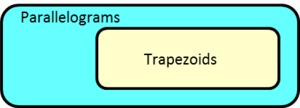# What is a Trapezoid? (Part 2)

Alignments to Content Standards: 5.G.B.4

Niko and Carlos are studying parallelograms and trapezoids. They agree that a parallelogram is a quadrilateral with two pairs of parallel sides. Niko says,

A trapezoid has one pair of parallel sides and a parallelogram has two pairs of parallel sides. So a parallelogram is also a trapezoid.

Carlos says,

No - a trapezoid can have only one pair of parallel sides.

Niko says,

That’s not true. A trapezoid has at least one pair of parallel sides, but it can also have another.

1. With a partner, discuss the difference between Niko’s definition and Carlos’ definition for a trapezoid.
2. Some people use Niko’s definition for a trapezoid, and some people use Carlos’ definition. Which statements below go with Niko’s definition? Which statements go with Carlos’ definition?
1. All parallelograms are trapezoids.
2. Some parallelograms are trapezoids.
3. No parallelograms are trapezoids.
4. All trapezoids are parallelograms.
5. Some trapezoids are parallelograms.
6. No trapezoids are parallelograms.
3. Which picture represents the relationship between trapezoids and parallelograms for each definition?
1.2.3.## IM Commentary

The purpose of this task is for students to compare different definitions for trapezoids. Some people define trapezoids to have one and only one pair of opposite sides parallel, while some people define trapezoids to have at least one pair of opposite sides parallel. The question of whether parallelograms are trapezoids depends on which of these definitions we agree on. Because of the care students need to take with definitions, this task draws heavily on MP6, Attend to precision. Most common quadrilaterals have a universally agreed upon definition: * Parallelograms are quadrilateral with 2 pairs of opposite parallel sides. * Rectangles are quadrilaterals with four right angles. * Rhombuses are quadrilaterals with equal side lengths. * Squares are quadrilaterals with equal side lengths and four right angles. The situation with trapezoids is somewhat unusual, but it gives students a chance to understand that definitions are conventions and for this reason we need to reason from agreed-upon definitions rather than shape recognition alone. While both definitions are legitimate, the benefit of Niko's definition (sometimes called "the inclusive definition") is that any theorem proved true for a trapezoid is also true for a parallelogram. Furthermore, in their study The Classification of Quadrilaterals (Information Age Publishing, 2008), Usiskin et al. conclude,

The preponderance of advantages to the inclusive definition of trapezoid has caused all the articles we could find on the subject, and most college-bound geometry books, to favor the inclusive definition.

The inclusive definition sets up a relationship between parallelograms and trapezoids that is exactly analogous to to the relationship between squares and rectangles; the definition for rectangles includes squares in the same way that the inclusive definition of trapezoids includes parallelograms. Please see the K-6 Geometry Progressions document for more information about these issues: http://commoncoretools.me/wp-content/uploads/2012/06/ccss_progression_g_k6_2012_06_27.pdf.

## Attached Resources

• Lesson Plan: Plane Figure Court
• ## Solution

1. The main difference is that Niko's definition includes parallelograms but Carlos' definition doesn't.
2. These statements go with Niko’s definition:

1. All parallelograms are trapezoids.
2. Some trapezoids are parallelograms.

These statements go with Carlos' definition:

1. No parallelograms are trapezoids.
2. No trapezoids are parallelograms.

3. Niko's definition goes with the first picture and Carlos' definition goes with the second picture.